CATEGORIES:

# Rank of a Matrix

Definition. Consider two systems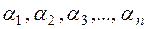;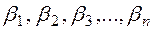.

These systems are said to be linearly dependent ifwhere k1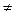0 or k20.

If these relations hold only for k1=0 and k2=0, then these two systems are linearly independent.

Consider anmatrix.

Definition. The maximal number of linearly independent rows in the matrix А is called the rank of this matrix.

The maximal number of linearly independent rows equals the maximal number of linearly independent columns.

Definition. A kth-order minor of a matrix А is the determinant consisting of the elements of arbitrarily chosen k columns and k rows.

Theorem. If all kth-order minors of a matrix are zero, then all (k+j)th-order minors are also zero.

Theorem. The rank of a matrix equals the maximal order of a nonzero minor.

The first method for calculating the rank of matrix (the bordering method).

(а) The method is to pass lower-order minors to higher-orders minors.

(b) Choose a nonzero minor and border it by a column and a row.

(c) If all of the bordered minors are zero, then the rank of the matrix equals the order of the nonzero kth-order minor:.

Example . Calculate the rank of a matrix.

We have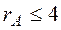, i.e., can the rank not be larger than 4.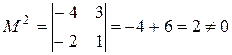,,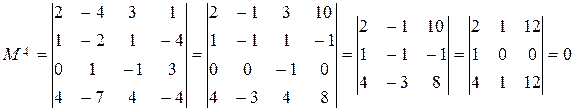,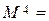.

Since all of forth-order minors equal zero and the determinant of third order does not equal zero, it follows that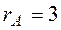.

The second method for calculating the rank of a matrix.

The elementary transformations of matrices include:

- permutation of columns or rows in a matrix,

- multiplication and division of the elements of a row or a column by a nonzero number,

- linear combination of rows (columns).

The elementary transformations of a matrix do not affect its rank.

Theorem. The rank of a matrix equals the number of nonzero elements on the main diagonal of any diagonal matrix obtained by elementary transformations from the initial matrix. (Without proof).

In the Gauss-Jordan method, only operations on rows are allowed; in calculating rank, we may operate on both rows and columns.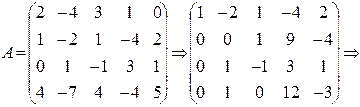We interchanged the first and second rows and obtained zeroes in the first column by using the second row. Then, using the first column, which has only one nonzero element, we obtained zeroes in the first row. Interchanging the second and third rows and using the new second row, we obtain zeroes in the second column, etc.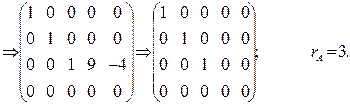Kronecker-Capelli theorem. Consider the system of m linear equations with n unknowns:(8)

Let us compose the principal and augmented matrices of this system: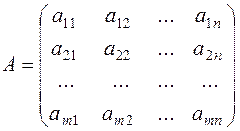;.

Theorem. A system of equations is consistent if and only if the rank of the augmented matrixequals that of the principal matrix А, i.e.,. (9)

Corollary 1. Any homogeneous system of equationsis consistent. Indeed, it suffices to take bi=0 for all i.

Corollary 2. System of equations (8) is consistent and has a unique solution if and only if the ranks of the matrices are equal and coincide with the number of unknowns.

Example. Determine whether the following system is consistent: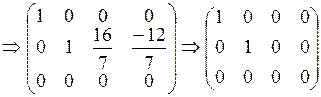,,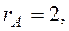The system is inconsistent.

Date: 2015-01-02; view: 1815

 <== previous page | next page ==> The Gauss-Jordan Method | Inverse Matrices
doclecture.net - lectures - 2014-2023 year. Copyright infringement or personal data (0.01 sec.)